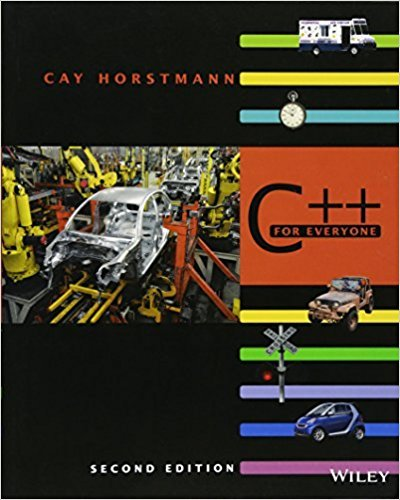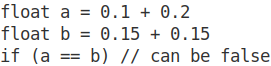×
Get Full Access to C++ For Everyone - 2 Edition - Chapter 3 - Problem R3.5
Get Full Access to C++ For Everyone - 2 Edition - Chapter 3 - Problem R3.5

×

# Explain why it is more difficult to compare floating-point numbers than integers.WriteISBN: 9780470927137 356

## Solution for problem R3.5 Chapter 3

C++ for Everyone | 2nd Edition

• Textbook Solutions
• 2901 Step-by-step solutions solved by professors and subject experts
• Get 24/7 help from StudySoup virtual teaching assistantsC++ for Everyone | 2nd Edition

4 5 1 257 Reviews
20
5
Problem R3.5

Explain why it is more difficult to compare floating-point numbers than integers.Write C++ code to test whether an integer n equals 10 and whether a floating-pointnumber x equals 10

Step-by-Step Solution:

R3.5 Explain why it is more difficult to compare floating-point numbers than integers. Write C++ code to test whether an integer n equals 10 and whether a floating-point number x equals 10.

Step By Step Solution

Step 1 of 4

Comparing two integers is very easy. Integer comparison is well defined and works on any integer value. However, even an operation seemingly as simple as comparing two floating-point numbers can cause trouble.

Floating-point numbers only have limited precision so it’s very likely that any mathematical operation (like addition, multiplication, etc.) will introduce small errors into the result. Also due to rounding errors, most floating point numbers end up being slightly imprecise. As long as this imprecision stays small, it can usually be ignored. However, it also means that numbers expected to be equal (e.g. when calculating the same result through different correct methods) often differ slightly, and a simple equality test fails. For example:Step 2 of 4

Step 3 of 4

##### ISBN: 9780470927137

This full solution covers the following key subjects: . This expansive textbook survival guide covers 10 chapters, and 515 solutions. This textbook survival guide was created for the textbook: C++ for Everyone , edition: 2. C++ for Everyone was written by and is associated to the ISBN: 9780470927137. The answer to “Explain why it is more difficult to compare floating-point numbers than integers.Write C++ code to test whether an integer n equals 10 and whether a floating-pointnumber x equals 10” is broken down into a number of easy to follow steps, and 29 words. The full step-by-step solution to problem: R3.5 from chapter: 3 was answered by , our top Engineering and Tech solution expert on 03/08/18, 08:39PM. Since the solution to R3.5 from 3 chapter was answered, more than 421 students have viewed the full step-by-step answer.

## Discover and learn what students are asking

Statistics: Informed Decisions Using Data : Inference about the Difference between Two Medians: Dependent Samples
?In Problems 3–10, use the Wilcoxon matched-pairs signedranks test to test the given hypotheses at the a = 0.05 level of significance. The dependent sa

Unlock Textbook Solution